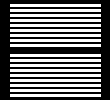# PHP LOOPS

 This Lesson displays different php loops and how to incorporate them into a GD script. It does not cover all types of loops but but it is a good place to begin. You do not need to ccp anything on this page. All the scripts are provided in this ZIP file Aforeach loop loops through an array. Set your variables into an array. Within the foreach statement, give the array an alias (a variable not declared elsewhere). PHP GD "; } ?> Will output: red green blue \$red=imagecolorallocate(\$image, 255,0,0); \$green=imagecolorallocate(\$image, 0,255,0); \$blue=imagecolorallocate(\$image, 0,0,255); imagesetthickness(\$image,4); \$i=0; \$colours = array(\$red, \$green, \$blue); foreach(\$colours as \$shade) { \$i+=10; imageline(\$image, 10,\$i,100,\$i,\$shade); } Will output: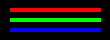For Loop \$x=0; while(\$x<=75) { echo "X=\$x,"; \$x+=15; } Above will output: X=0,X=15,X=30,X=45,X=60,X=75 \$x=0; \$i=10; while(\$x<=75) { \$x+=15; \$i+=4; imageline(\$image, 0,\$i,\$x,\$i,\$white); }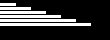For the GD script I had to add another integer (\$i) to get the lines to move down the page. For some reason this does start drawing at 0, as opposed to 15. Maybe because I used \$x=\$x+15 in the last lesson. For Loop with Even/Odd exception -- else clause for(\$i = 0; \$i <= 10; \$i++) { if(\$i % 2 == 0) { echo "\$i is even
"; } else { echo "\$i is odd%lt;br>"; } } This will output: 1 is odd 2 is even 3 is odd 4 is even 5 is odd 6 is even 7 is odd 8 is even 9 is odd 10 is even This loop also throws an exception into it with the else{} clause. If \$i is divisible by 2 with no decimal, it is even. imagesetthickness(\$image,3); \$x=0; for(\$i = 0; \$i <= 10; \$i++) { if(\$i % 2 == 0) { \$x+=2; imageline(\$image, 0,\$i+\$x,50,\$i+\$x,\$white); } else { \$x+=2; imageline(\$image, 0,\$i+\$x,50,\$i+\$x,\$red); } }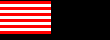The percent sign is used for division as opposed to a / . If I used a / it would have returned a decimal number. See php math operators for more details. PHP operators For Loop with a break -- if statement for(\$a = 0; \$a < 10; \$a++){ print \$a . "
"; if(\$a == 5){ break; } } This loop prints 0 1 2 3 4 5 \$x=0; for(\$a = 0; \$a < 200; \$a++){ imageline(\$image, 0,10,\$a,10,\$white); if(\$a == 100){ break; } }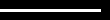I threw this loop in to show how to break out of a loop. Sometimes you can make an error and get stuck waiting and waiting while the page is stuck in an infinite loop. On this example, the page width = 110, so I wanted to stop the loop before it got off the page. Might be a good exception to throw into a loop you are trying to figure out. You can always remove the if statement if the loop works right. For Loop with a continue statement -- if statement for(\$a = 0; \$a <= 10; \$a++){ if(\$a == 5){ continue; } print \$a . "
"; } This loop prints out 0 1 2 3 4  6 7 8 9 10 The if statement here tells the loop to skip number 5 and continue with the loop to the end. for(\$a = 5; \$a < 100; \$a+=5){ if(\$a == 55){ continue; } imageline(\$image, 10,\$a,100,\$a,\$white); }